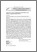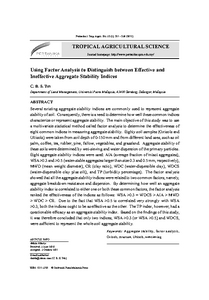# Using factor analysis to distinguish between effective and ineffective aggregate stability indices

## Citation

Teh, Christopher Boon Sung (2012) Using factor analysis to distinguish between effective and ineffective aggregate stability indices. Pertanika Journal of Tropical Agricultural Science, 35 (3). pp. 521-536. ISSN 1511-3701; ESSN: 2231-8542

## Abstract

Several existing aggregate stability indices are commonly used to represent aggregate stability of soil. Consequently, there is a need to determine how well these common indices characterize or represent aggregate stability. The main objective of this study was to use a multivariate statistical method called factor analysis to determine the effectiveness of eight common indices in measuring aggregate stability. Eighty soil samples (Oxisols and Ultisols) were taken from soil depth of 0-150 mm and from different land uses, such as oil palm, coffee, tea, rubber, pine, fallow, vegetables, and grassland. Aggregate stability of these soils were determined by wet-sieving and water dispersion of the primary particles. Eight aggregate stability indices were used: AIA (average fraction of intact aggregates), WSA >0.3 and >0.5 (water-stable aggregates larger than size 0.3 and 0.5 mm, respectively), MWD (mean weight diameter), CR (clay ratio), WDC (water-dispersible clay), WDCS (water-dispersible clay plus silt), and TP (turbidity percentage). The factor analysis showed that all the aggregate stability indices were related to two common factors, namely, aggregate breakdown resistance and dispersion. By determining how well an aggregate stability index is correlated to either one or both these common factors, the factor analysis ranked the effectiveness of the indices as follows: WSA >0.3 = WDCS > AIA > MWD > WDC > CR. Due to the fact that WSA >0.5 is correlated very strongly with WSA >0.3, both the indices ought to be as effective as the other. The TP index, however, had a questionable efficacy as an aggregate stability index. Based on the findings of this study, it was therefore concluded that only two indices, WSA >0.3 (or WSA >0.5) and WDCS, were sufficient to represent the whole soil aggregate stability.Preview
PDF
60. Using factor analysis to distinguish between effective and ineffective aggregate stability indices.pdfView Item